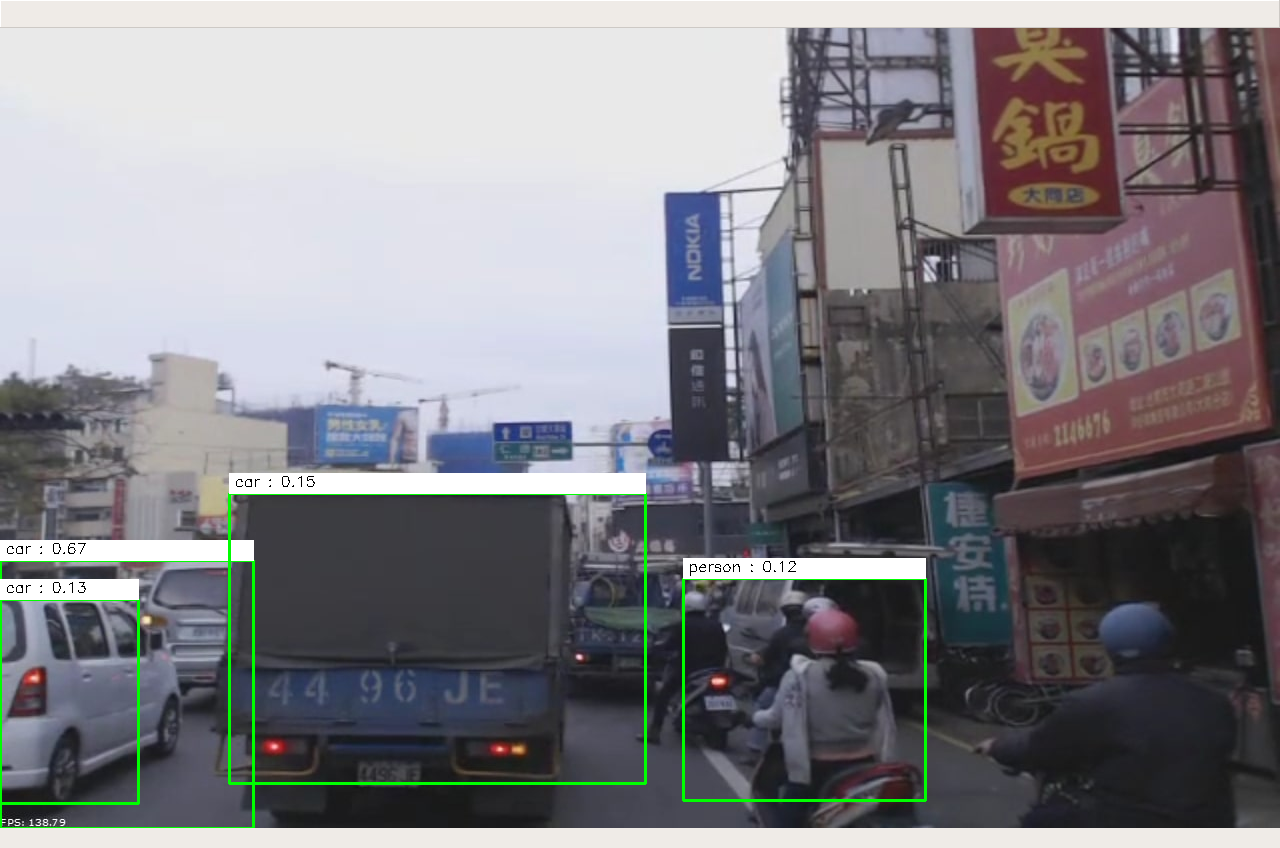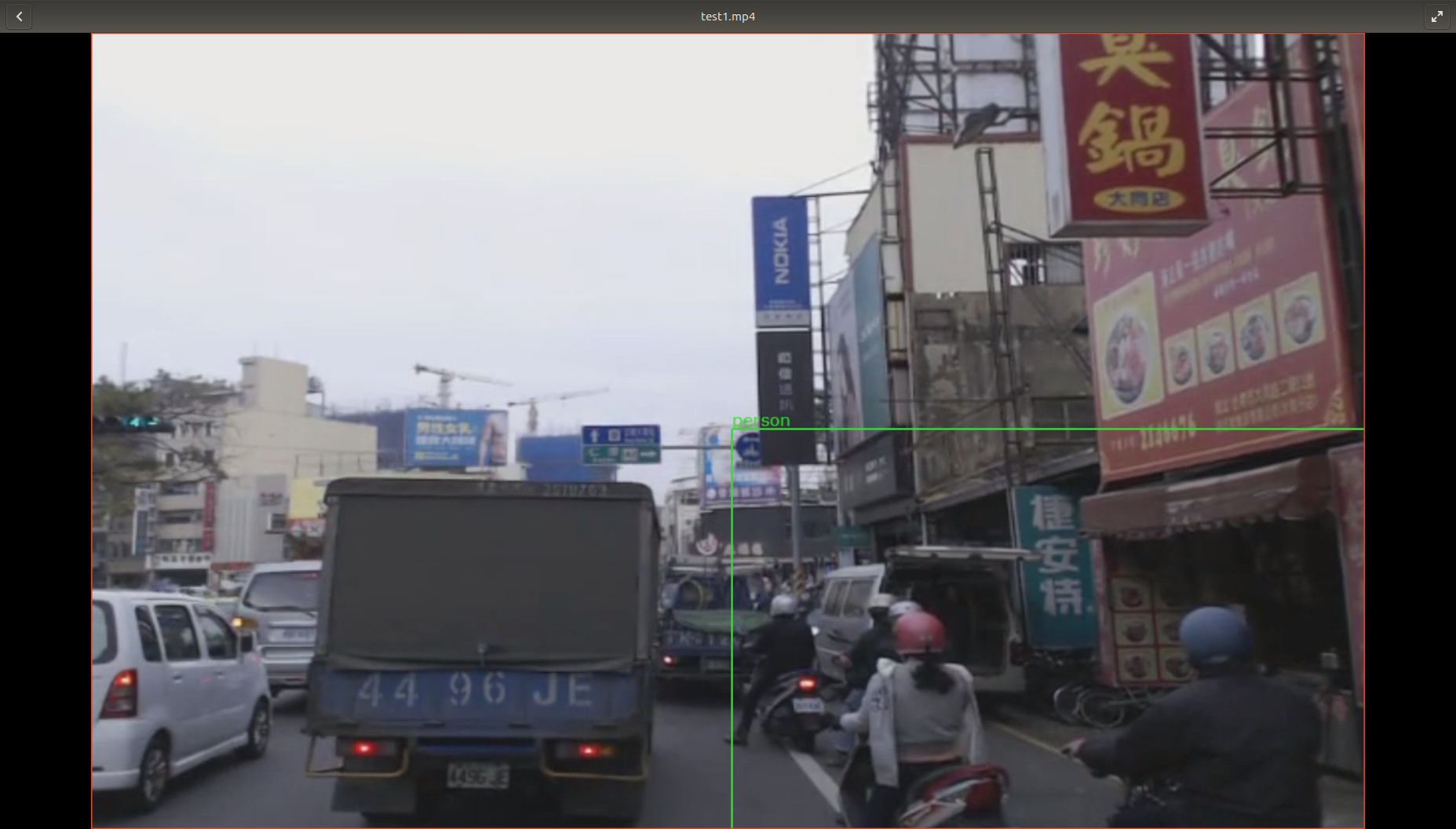# YOLO TensorRT model on sample_object_detector with weird bounding boxes

HI!
I already performed the YOLO tendorRT model on sample_object_detector but the bounding boxes seemed so weird. I’m sure that the original YOLO model was trained and is good enough to predict bounding box.
Below is the reference results on caffe-yolo: https://github.com/xingwangsfu/caffe-yolo/I referred the caffe-yolo and modified sample_object_detector to do the reference.
Below is the screenshots of my result.The bounding boxes are mess around…
My modification is mainly about the function interpretOutput in sample_object_detector.
Below is my source code.

``````void interpretOutput(const float32_t *outBBox, const dwRect *const roi)
{
m_detectedBoxList.clear();
m_bboxConfList.clear();
m_boxLabelList.clear();
std::string classes = {"aeroplane", "bicycle", "bird", "boat", "bottle", "bus", "car", "cat", "chair", "cow", "diningtable", "dog", "horse", "motorbike", "person", "pottedplant", "sheep", "sofa", "train","tvmonitor"};
float threshold = 0.02;//yolo-small
//float iou_threshold = 0.5;
int num_class = 20, num_box = 2, grid_size = 7;
float probs[grid_size][grid_size][num_box][num_class] = {0};
float class_probs[grid_size][grid_size][num_class] = {0};
float scales[grid_size][grid_size][num_box] = {0};
float boxes[grid_size][grid_size][num_box] = {0};
matrix<int> filter_boxes(m_maxDetections, 4);
matrix<double> filtered_boxes(m_maxDetections, 4);
vector<double> filtered_probs(m_maxDetections);
int t = 0, i = 0, j = 0, k = 0, l = 0, detected_num = 0;
for(i = 0; i < grid_size; i++){
for(j = 0; j < grid_size; j++){
for(k = 0; k < num_class; k++){
class_probs[i][j][k] = outBBox[t];
t = t + 1;
}
}
}
for(i = 0; i < grid_size; i++){
for(j = 0; j < grid_size; j++){
for(k = 0; k < num_box; k++){
scales[i][j][k] = outBBox[t];
t = t + 1;
}
}
}
for(i = 0; i < grid_size; i++){
for(j = 0; j < grid_size; j++){
for(k = 0; k < num_box; k++){
for(l = 0; l < 4; l++){
boxes[i][j][k][l] = outBBox[t];
t = t + 1;
}
}
}
}
//Find matching boxes and note down the location
int g_y = 0, g_x = 0;
for(g_y = 0; g_y < grid_size; g_y++){
for(g_x = 0; g_x < grid_size; g_x++){
for(i = 0; i < num_box; i++){
boxes[g_y][g_x][i] = ((boxes[g_y][g_x][i] + g_x) / grid_size) * roi->width;
boxes[g_y][g_x][i] = ((boxes[g_y][g_x][i] + g_y) / grid_size) * roi->height;
boxes[g_y][g_x][i] = pow(boxes[g_y][g_x][i], 2) * roi->width;
boxes[g_y][g_x][i] = pow(boxes[g_y][g_x][i], 2) * roi->height;
for(j = 0; j < num_class; j++){
probs[g_y][g_x][i][j] = scales[g_y][g_x][i] * class_probs[g_y][g_x][j];
if(probs[g_y][g_x][i][j] >= threshold){
filter_boxes(detected_num, 0) = g_y;//y location
filter_boxes(detected_num, 1) = g_x;//x location
filter_boxes(detected_num, 2) = i;//one of the two predicted boxes
filter_boxes(detected_num, 3) = j;//which class
filtered_probs(detected_num) = probs[g_y][g_x][i][j];
detected_num += 1;
}
}
}
}
}
//Transfer the boxes' grid locations to the coordinates
int d = 0;
for(d = 0; d < detected_num; d++){
filtered_boxes(d, 0) = boxes[filter_boxes(d, 0)][filter_boxes(d, 1)][filter_boxes(d, 2)];//x
filtered_boxes(d, 1) = boxes[filter_boxes(d, 0)][filter_boxes(d, 1)][filter_boxes(d, 2)];//y
filtered_boxes(d, 2) = boxes[filter_boxes(d, 0)][filter_boxes(d, 1)][filter_boxes(d, 2)];//w
filtered_boxes(d, 3) = boxes[filter_boxes(d, 0)][filter_boxes(d, 1)][filter_boxes(d, 2)];//h
}
//Detect overlap boxes(equal to do NMS)
for(i = 0; i < detected_num; i++){
if(filtered_probs(i) == 0){
continue;
}
for(j = i + 1; j < detected_num; j++){
dwRectf objABox;
dwRectf objBBox;
objABox.x = filtered_boxes(i, 0);
objABox.y = filtered_boxes(i, 1);
objABox.width = filtered_boxes(i, 2);
objABox.height = filtered_boxes(i, 3);
objBBox.x = filtered_boxes(j, 0);
objBBox.y = filtered_boxes(j, 1);
objBBox.width = filtered_boxes(j, 2);
objBBox.height = filtered_boxes(j, 3);
float32_t ovl = overlap(objABox, objBBox);
float32_t iou = ovl / (objABox.width * objABox.height + objBBox.width * objBBox.height - ovl);
if(iou > m_nonMaxSuppressionOverlapThreshold){
filtered_probs(j) = 0.0;
}
}
}
//load detected boxes into the m_detectedBoxList vector
dwRectf bbox;
for(i = 0; i < detected_num; i++){
if(filtered_probs(i) != 0.0){
bbox.x = filtered_boxes(i, 0);
bbox.y = filtered_boxes(i, 1);
bbox.width  = filtered_boxes(i, 2);
bbox.height = filtered_boxes(i, 3);
m_detectedBoxList.push_back(bbox);
m_boxLabelList.push_back(classes[filter_boxes(i, 3)]);
m_bboxConfList.push_back(std::make_pair(bbox, filtered_probs(i)));
}
}
}
``````

Can someone give me some hints or some reasons?
Is that something about the using of tensorRT_optimization?

Hi,

Do you use the same output interpretation function in the training validation?
If no, could you check it on the training environment first?

Thanks.